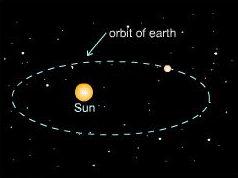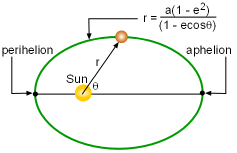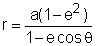Ch 11. Polar Coordinates Multimedia Engineering Math PolarCoordinates Area andLength ConicSections
 Chapter 1. Limits 2. Derivatives I 3. Derivatives II 4. Mean Value 5. Curve Sketching 6. Integrals 7. Inverse Functions 8. Integration Tech. 9. Integrate App. 10. Parametric Eqs. 11. Polar Coord. 12. Series Appendix Basic Math Units Search eBooks Dynamics Fluids Math Mechanics Statics Thermodynamics Author(s): Hengzhong Wen Chean Chin Ngo Meirong Huang Kurt Gramoll ©Kurt GramollMATHEMATICS - CASE STUDY IntroductionProblem DiagramAphelion and Perihelion Distance between the Earth and the Sun During a field trip to a national astrology center, a park ranger posed a challenging question to the 12th graders visiting the center. The earth was closest to the sun on that day, and he wanted to know what is the shortest (i.e., perihelion distance) and longest distance (i.e., aphelion distance) between the earth and the sun. It is known that the elliptical orbit of earth around the sun in polar coordinate is:where e is the eccentricity and a is the distance of the major axis. Questions Derive the equations for the perihelion distance and aphelion distance between the earth and the sun. What is the closest and farthest distance between the earth and the sun? Approach The eccentricity is approximately 0.02 and the distance of the major axis is 3 x 108 km.